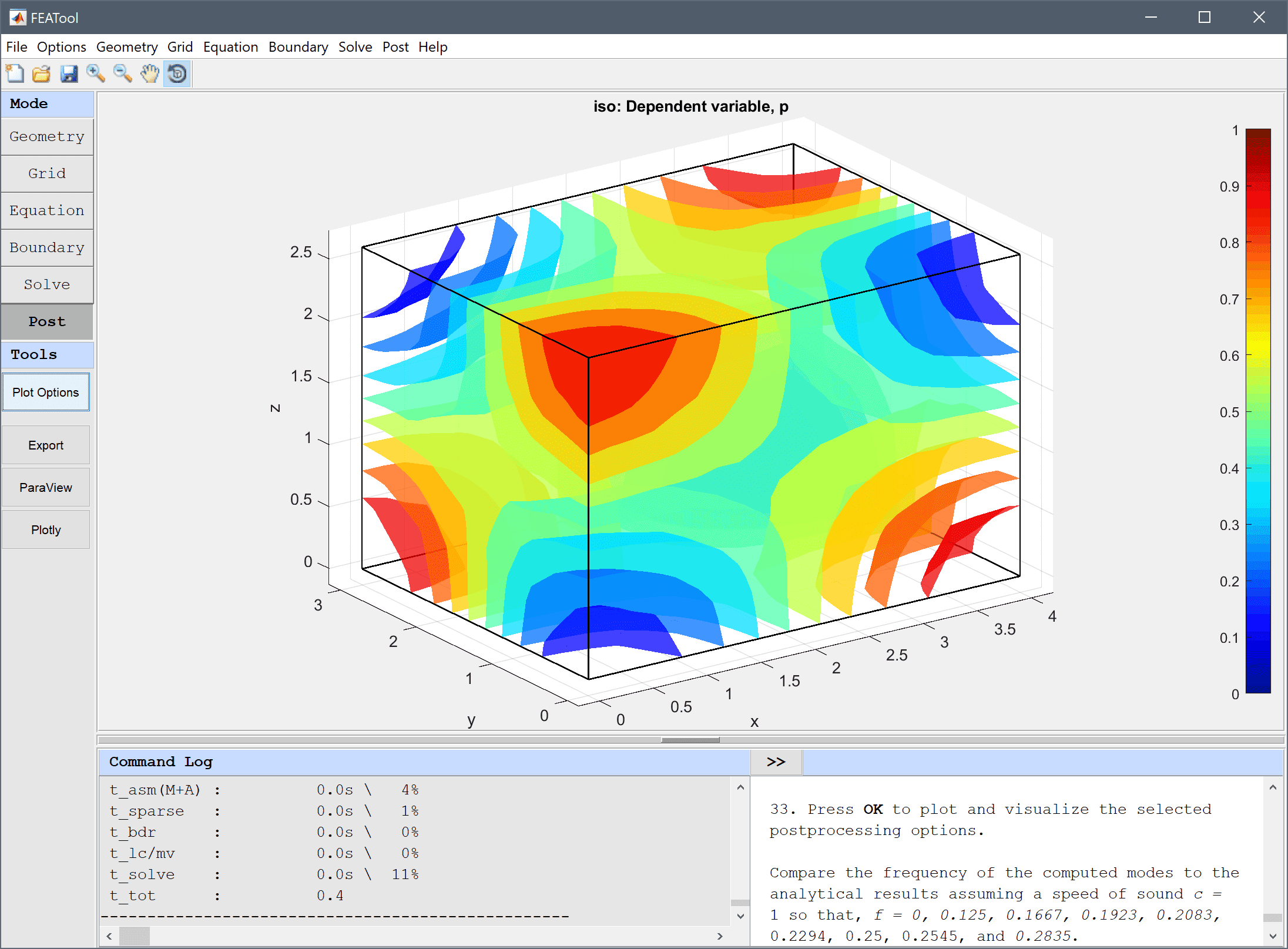# Resonance Frequencies of a Room## Model Data

Type: classic pde

Physics Modes: custom equation

This example studies the resonance frequencies of an empty room by using the Helmholz equation for the time-harmonic pressure field

$$\Delta p + k^2 p = 0$$

The resulting eigenmodes can be compared to and validated against the analytical solution for the resonance frequencies of a boxed enclosure, that is f = c/2*√( (i/lx)2 + (j/ly)2 + (k/lz)2 ) where i, j, and k are integer permutations 0 … n for the various modes.

This model is available as an automated tutorial by selecting Model Examples and Tutorials… > Classic PDE > Resonance Frequencies of a Room from the File menu. Or alternatively, follow the linked step-by-step instructions.